Next: Conclusion Up: Examples Previous: Numerical simulation

##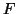-tests

Assume that for a set of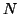subjects we model their hæmodynamic response functions using the same set of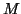basis functions for each subject and consider the case where we wish to test for the population mean activation. Thus, we implicitly assume that there exists a single hæmodynamic response function that is representative for the group activation. We will not restrict the choice of basis functions (i.e. we do not require the basis functions to be orthogonal) and therefore allow for general correlations between the individual basis funtion fits, but assume that the covariance structure is the same for each individual. That is, we model the subject-specific vector of fits as distributed according to a multivariate normal distribution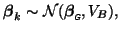and let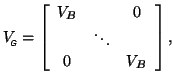where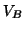is the covariance matrix of thebasis function fits. Then the group-level design matrixcombines theindividual basis functions across subjects such that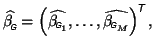where the individual values are themean basis coefficients. In order to assess the average population activation, we need to test if any of the basis function coefficients are significantly non-zero. This can be achieved by calculating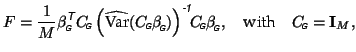which approximately follows an-distribution. If, instead, we wish to assess if the final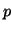basis functions contribute significantly to the mean fit, we simply set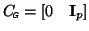and changeto.Next: Conclusion Up: Examples Previous: Numerical simulation
Christian Beckmann 2003-07-16